Function Repository Resource:

# Biarc

Generate a biarc through two points

Contributed by: Jan Mangaldan
 ResourceFunction["Biarc"][p1,d1,p2,d2] represents the biarc through the points p1 and p2, with corresponding tangent directions d1 and d2.

## Details

A biarc is a smooth curve formed from two circular arcs, whose continuity is imposed by making the two arcs have the same tangent at the point where they meet.
ResourceFunction["Biarc"] produces a JoinedCurve object which can be used in Graphics (two-dimensional graphics).
ResourceFunction["Biarc"][p1,d1,p2,d2,"Circle"] gives a list of Circle objects that comprise the biarc.

## Examples

### Basic Examples (1)

Show a biarc through two points, with given tangent directions:

 In:=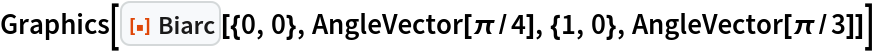Out=### Scope (2)

Show biarcs with varying tangent directions:

 In:=Out=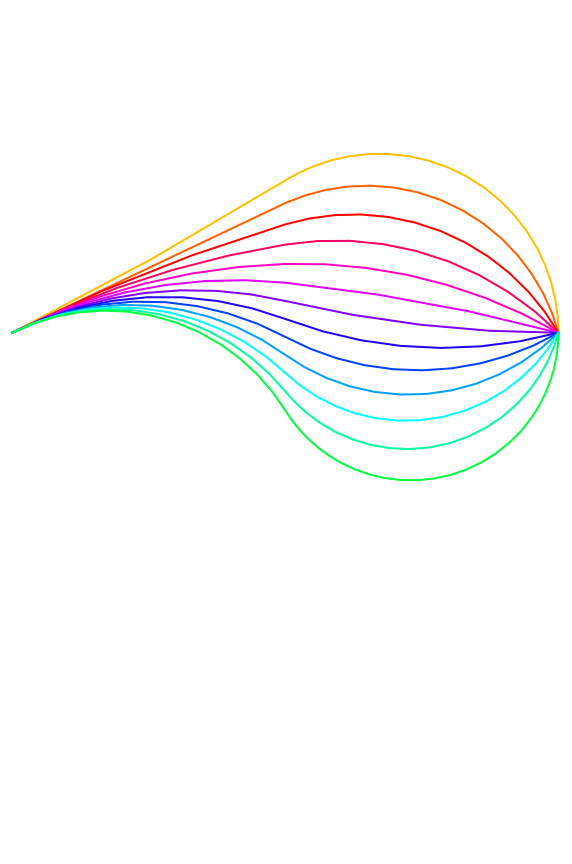Give the circle arcs comprising a biarc:

 In:=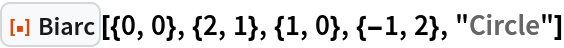Out=Show the circle arcs and their corresponding centers along with the biarc:

 In:=Out=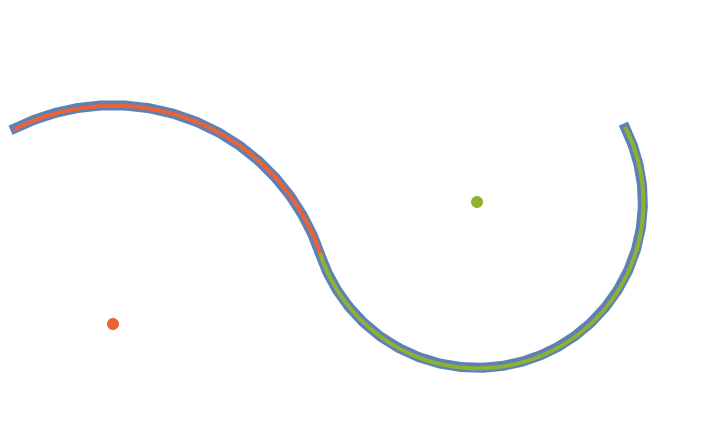### Applications (1)

Interactively manipulate a biarc:

 In:=Out=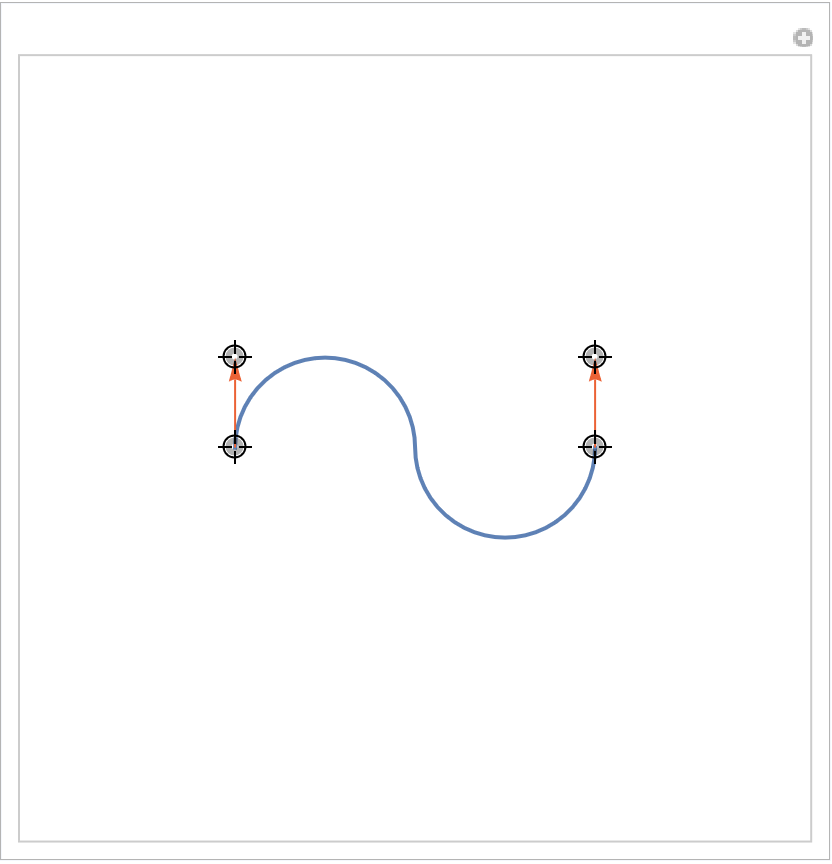### Properties and Relations (1)

Biarc gives a JoinedCurve object:

 In:=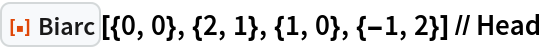Out=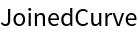## Version History

• 1.0.0 – 23 June 2022